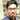# Bitwise Can Avoid Loops

January 30, 2012

As we have seen before, the power of bitwise operators are immense. Sometimes thinking in terms of arithmetical operators cost you lots of CPU time when compared to bitwise operators. So given a problem its obvious for us to think in terms of arithmetical solutions, but try to search for a bitwise solution and compare it with previous one. You can end up in a very powerful algorithm too. Now I wish to share one such case where the use of bitwise operator avoids the use of a loop too.

Problem Statement: Given a number, check whether its a positive power of two or not.

First I would like to give a simple arithmetical solution.

This is quite a good algorithm of order O(log2n), that is, given a number n, it will take log2n operations to find the result. So, if n is 256, then 8 operations are required to produce the result. The algorithm that we have come up with works at an amazing rate. O(log2n) is a very good algorithm. Still, if it can be reduced further to O(1) it will be great isn’t it. Bitwise operators come to the rescue.

Now see the program below which is the bitwise algorithm for solving the same problem.

This bitwise algorithm has only one operation which checks a & (a-1) is 0 or not. If its 0, then its a power of 2 otherwise its not. How? It seems amazing isn’t it? Let’s see.

First note the binary representations of the powers of 2.

``````2^0 =   1 => 00000001
2^1 =   2 => 00000010
2^2 =   4 => 00000100
2^3 =   8 => 00001000
2^4 =  16 => 00010000
2^5 =  32 => 00100000
2^6 =  64 => 01000000
2^7 = 128 => 10000000``````

Now check out the other part, binary representations of (2^n)-1.

``````(2^0)-1 =   0 => 00000000
(2^1)-1 =   1 => 00000001
(2^2)-1 =   3 => 00000011
(2^3)-1 =   7 => 00000111
(2^4)-1 =  15 => 00001111
(2^5)-1 =  31 => 00011111
(2^6)-1 =  63 => 00111111
(2^7)-1 = 127 => 01111111``````

A sample and operation of a power of 2 and its previous number.

``8&7 => 00001000 & 00000111 => 00000000``

And when its not a power of 2

``6&5 => 00000110 & 00000101 => 00000100``

Bitwise representation of a number which is power of 2 varies at every bit from the previous numbers bitwise representation. But when its not a power of two, they have at least one ‘1’ in common position. Now its clear that whenever you make an AND operation on the numbers which is power of 2 and one less than that, ultimately you end up at zero. Similarly, if the number is not a power of 2, you will end up in a non zero number.

This is an example to show the power of bitwise operators. Hope you enjoyed.Written by Fareez Ahamed who lives and works in Chennai, India building useful things. You should follow him on Twitter